Forestry workers

In the forest is employed 56 laborers planting trees in nurseries. For 8 hour work day would end job in 37 days. After 16 days, 9 laborers go forth?

How many days are needed to complete planting trees in nurseries by others, if they will work 10 hours a day?

Result

x =  20 d

Solution:

56 * 8 * 37 = 16 * 8 * 56 + (56 - 9) * 10 * x

470x = 9408

x = 4704235 ≈ 20.017021

Calculated by our simple equation calculator.

Leave us a comment of this math problem and its solution (i.e. if it is still somewhat unclear...):Be the first to comment!To solve this verbal math problem are needed these knowledge from mathematics:

Need help calculate sum, simplify or multiply fractions? Try our fraction calculator. Do you have a linear equation or system of equations and looking for its solution? Or do you have quadratic equation?

Next similar math problems:

1. FactoryIn the factory workers work in three shifts. In the first inning operates half of all employees in the second inning and a third in the third inning 200 employees. How many employees work at the factory?
2. Log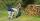Worker cut his thick log to 6 pieces for 30 min. How long he cut log to 12 pieces?
3. Pumps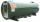6 pump fills the tank for 3 and a half days. How long will fill the tank 7 equally powerful pumps?
4. Cook on gas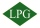The gas cylinder will last for 30 weekends for 2 hours of daily cooking. How many days will we be able to cook on a new cylinder when we cook 3 hours a day?
5. Tractors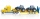5 tractors will plow the field for 8 hours. In 2 hours, 1 tractor broke down. How long do the remaining tractors complete the plow?
6. What isWhat is the value of the smaller of a pair of numbers for which their sum is 78 and their division quotients are 0.3?
7. The farmer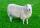The farmer had 140 sheep. For the next year, she decided to change the number of sheep in ratio 10: 7. How many sheep will he have then?
8. Write 2Write 791 thousandths as fraction in expanded form.
9. UN 1If we add to an unknown number his quarter, we get 210. Identify unknown number.
10. Number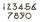What number I think? The third is 6 and half is 2 more than the its quarter.
11. Family and age ratiosAge of father and son are in the ratio 10:3; age of father and daughter are in the ratio 5:2. How old is father and son, if daughter is 20 years old? In what ratio is age sister and brother?
12. Fraction and a decimalWrite as a fraction and a decimal. One and two plus three and five hundredths
13. Bag of peanuts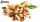Joe eat 1/3 of a bag of peanuts, mark eat 1/4 of the remaining in the bag of peanuts, Alvin eat 1/2 of the remaining bag of peanuts, peter eat 10 peanuts, there are 71 peanuts left. Hon many peanuts were in the bags?
14. Product of two fractionsProduct of two fractions is 9 3/5 . If one of the fraction is 9 3/7. Find the other fraction.
15. FractionsThree-quarters of an unknown number are 4/5. What is 5/6 of this unknown number?
16. Boys and girlsThere are 48 children in the sports club, boys are 10 more than girls. How many girls go to the club?
17. Football match 4In a football match with the Italy lost 3 goals with Germans. Totally fell 5 goals in the match. Determine the number of goals of Italy and Germany.